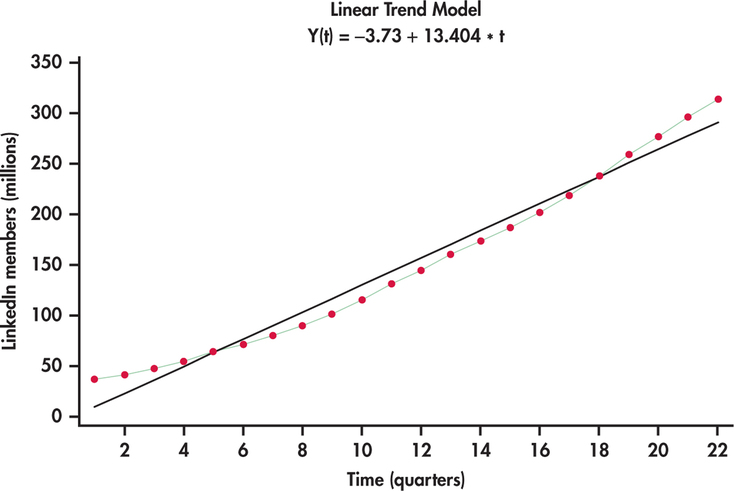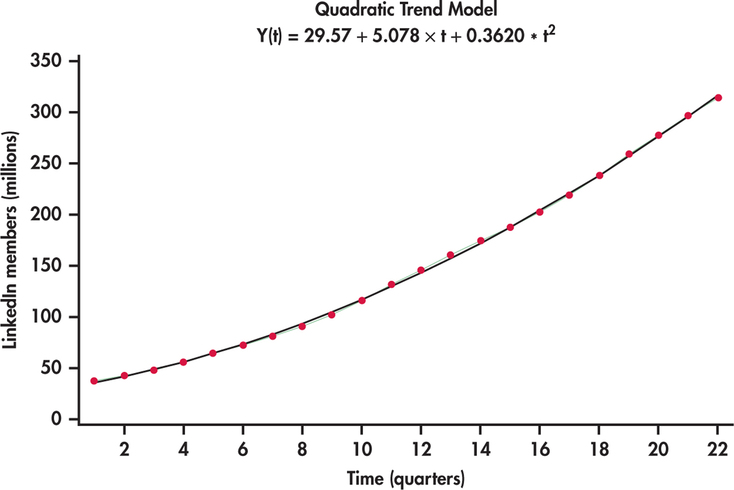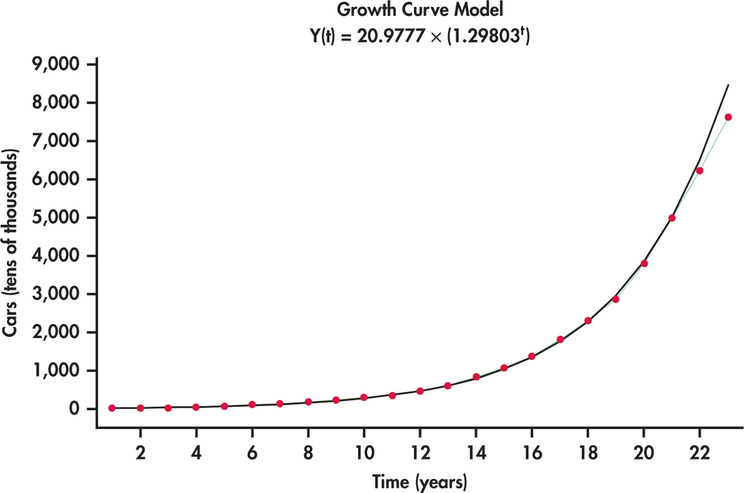## 15.2 Modeling the trend

• The simplest models for trend are regressions of the variable $$y$$ with respect to time..

• A linear trend model is:

$t_{t}=\alpha_{0}+\alpha_{1} t, \,\, \,\, \forall t=1,2, \ldots, T.$

The parameter $$\alpha_{1}$$ represents the slope and describes the expected growth between two periods. The forecast of the variable $$y$$ for the period $$T+k$$ is:

$\hat{y}_{T}(k)=\hat{\alpha}_{0}+\hat{\alpha}_{1}(T+k)$• A quadratic trend model is:

$t_{t}=\alpha_{0}+\alpha_{1} t+\alpha_{2} t^{2}, \,\,\,\, \forall t=1,2, \ldots, T.$• An exponential trend model is:

$t_t = \exp \left ( \alpha_0 +\alpha_1 t \right ) .$

By taking logarithms is:

$\log t_{t} = \alpha_{0} + \alpha_{1} t + \varepsilon_{t}$